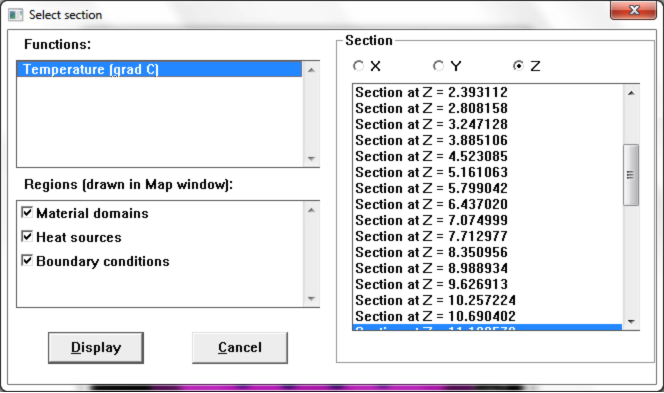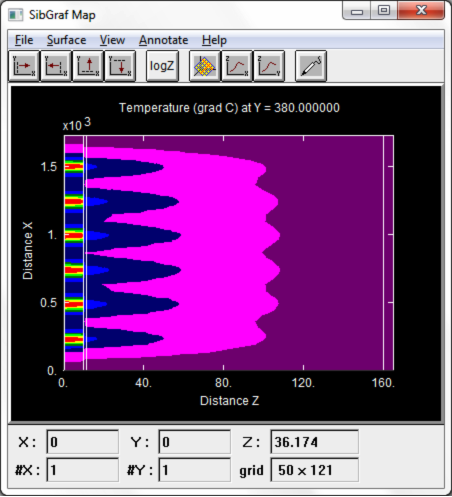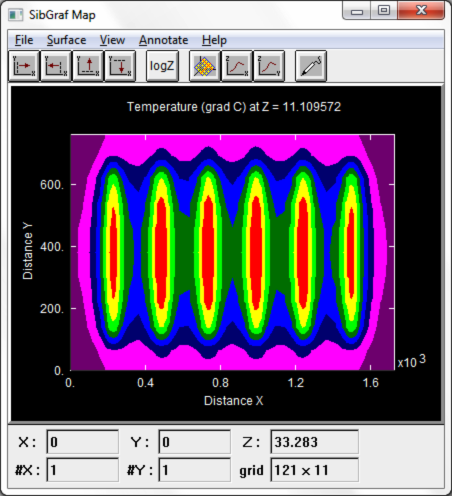# SibLin for Windows

## 3D Poisson/Diffusion/Heat Transfer Equation Solver

Licensed to Spectrian, Sunnyvale, California, Nortel, Ottawa, and Waterloo Hydrogeologic Inc, Ontario, Canada.

SibLin is a linear solver for matrices arising in 2D and 3D finite-difference solutions of various partial differential equations such as the Poisson equation, Heat Transfer equation, Diffusion equation etc. The method is based on the BiCGSTAB algorithm by H. Van der Vorst and solves both symmetric and non-symmetric matrices.

SibLin Graphical User Interface allows to plot two-dimensional distributions of the obtained solution at various cross-sections of the three-dimensional structure.The two-dimensional distributions in the cross-sections, in turn, can be further visualized by a one-dimensional cross-section in either direction in the two-dimensinal plane.